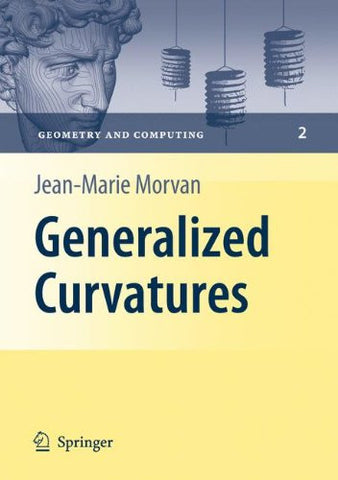Generalized Curvatures (Geometry and Computing, Vol. 2)

• \$56.16
• Save \$270
• Binding: Hardcover
• Author: Jean-Marie Morvan
• Publish Date: 2008-07-18

Attention : For textbook, access codes and supplements are not guaranteed with used items.

The central object of this book is the measure of geometric quantities describing N a subset of the Euclidean space (E ,), endowed with its standard scalar product. Let us state precisely what we mean by a geometric quantity. Consider a subset N S of points of the N-dimensional Euclidean space E , endowed with its standard N scalar product. LetG be the group of rigid motions of E . We say that a 0 quantity Q(S) associated toS is geometric with respect toG if the corresponding 0 quantity Q[g(S)] associated to g(S) equals Q(S), for all g?G . For instance, the 0 diameter ofS and the area of the convex hull ofS are quantities geometric with respect toG . But the distance from the origin O to the closest point ofS is not, 0 since it is not invariant under translations ofS. It is important to point out that the property of being geometric depends on the chosen group. For instance, ifG is the 1 N group of projective transformations of E , then the property ofS being a circle is geometric forG but not forG , while the property of being a conic or a straight 0 1 line is geometric for bothG andG . This point of view may be generalized to any 0 1 subsetS of any vector space E endowed with a groupG acting on it.